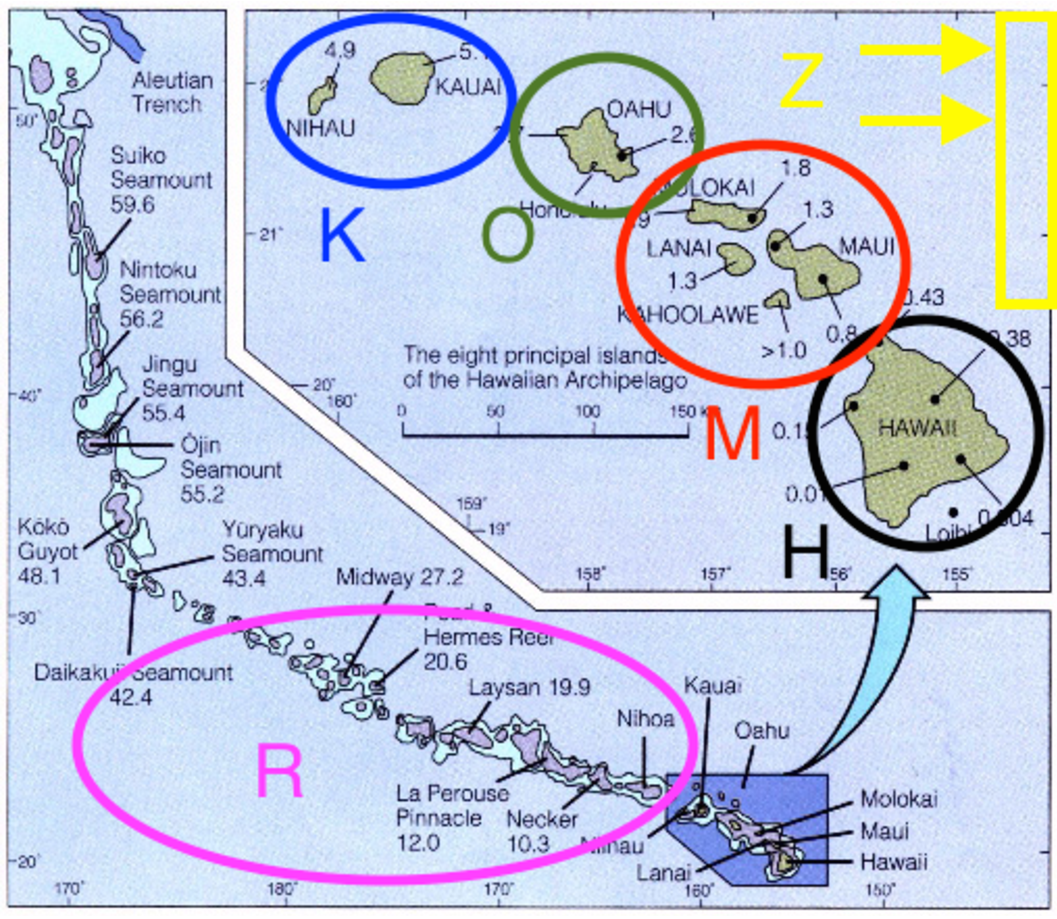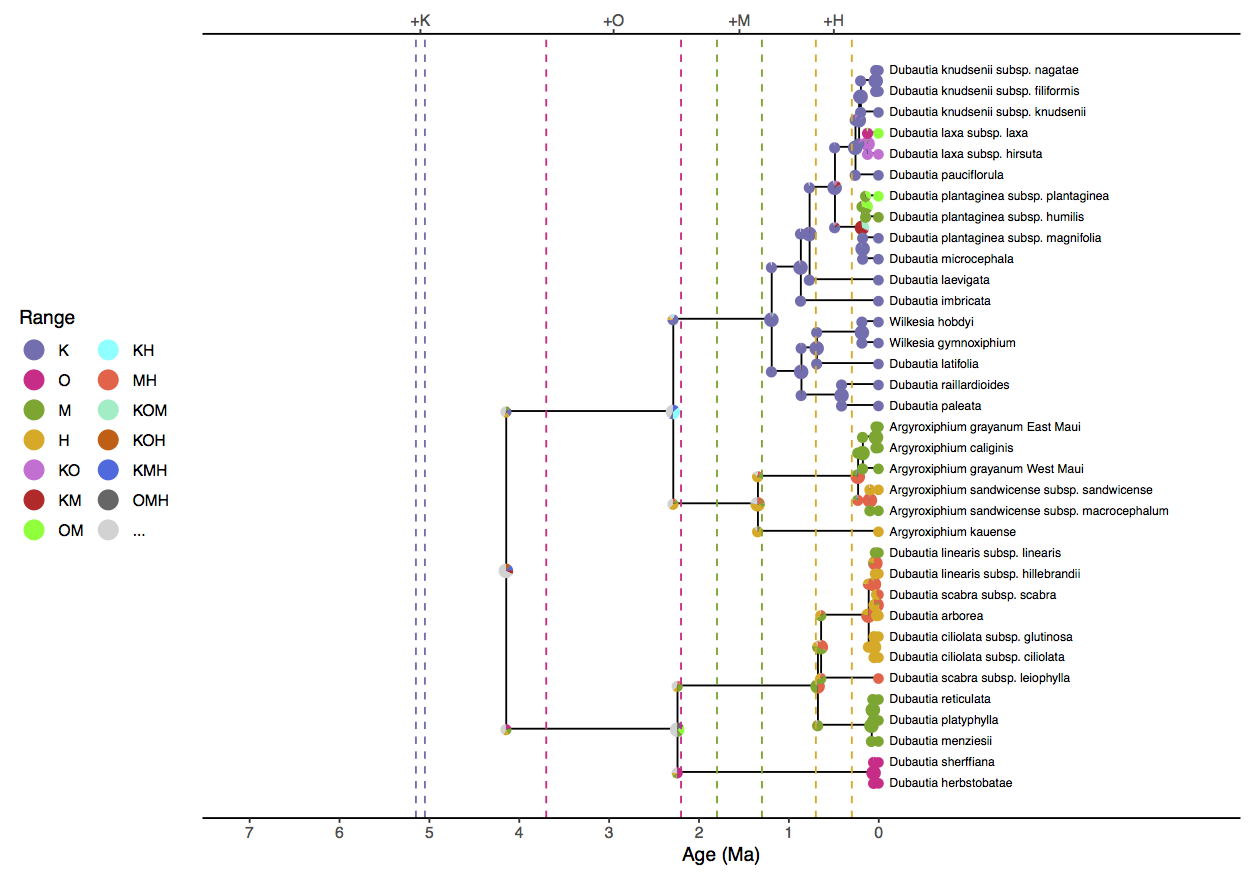# Simple Phylogenetic Analysis of Historical Biogeography

## Introduction

In the Introduction to Phylogenetic Models of Discrete Biogeography tutorial, we went through the exercise of setting up the instantaneous rate matrix and cladogenetic transition probabilities for a simple DEC model. In this tutorial, we will complete a biogeographic analysis using an empirical dataset: the Hawaiian silverswords.

## A Simple DEC Analysis

The following series of tutorials will estimate the ancestral ranges of the silversword alliance (Tribe Madiinae), a young and diverse clade of about 50 species and subspecies. Although silverswords are endemic to Hawaii, they are nested within a larger clade alongside tarweeds, which are native to western continental North America (Baldwin et al. 1991). The size and age of the silversword clade, combined with our knowledge of Hawaiian island formation, makes it an ideal system to explore concepts in historical biogeography and phylogeny. For further reading, consult Carlquist (1959) and Baldwin and Sanderson (1998).A beautiful figure of the discrete areas for the tutorial. Six areas are shown: Kauai and Niihau (K); Oahu (O); Maui-Nui, Lanai, and Molokai (M); Hawaii (H); the remaining Hawaiian islands (R); and the North American mainland (Z).

For this tutorial we’ll focus entirely on the silversword alliance and the modern Hawaiian archipelago. To begin, we’ll use just four areas, K, O, M, and H, and include areas R and Z in later analyses (). The species ranges used in this exercise follow Gillespie and Baldwin (2009).

### Getting Set Up

We have provided the following data files for this tutorial:

Once you have downloaded the zip file by clicking on the arrow above, Unzip that and navigate inside of that directory. This directory should be called revbayes_biogeo_biogeo_simple

Once inside you should see two directories: data and scripts

Once you have all of the files, open RevBayes and ensure that your working directory is the top directory above the data folder (e.g., revbayes_biogeo_biogeo_simple).

### Analysis

First, create file management variables for input and output

range_fn = "data/n4/silversword.n4.range.nex"
tree_fn = "data/n4/silversword.tre"
out_fn = "output/simple"


then read in our character data as binary presence-absence characters

dat_range_01 = readDiscreteCharacterData(range_fn)


then encode the species ranges into natural numbers

dat_range_n = formatDiscreteCharacterData(dat_range_01, "DEC")


Record the number of areas (characters) from the discrete character data object

n_areas = dat_range_01.nchar()


You can view the taxon data to see how characters are coded both as human-readable presence-absence data

dat_range_01

      Argyroxiphium_grayanum_East_Maui:
0010


dat_range_n

      Argyroxiphium_grayanum_East_Maui:
3


We’ll want to record the relationship between range states and range labels when producing an ancestral range estimate figure. First, store the vector of range state descriptions

state_desc = dat_range_n.getStateDescriptions()


then write it to file

state_desc_str = "state,range\n"
for (i in 1:state_desc.size()){
state_desc_str += (i-1) + "," + state_desc[i] + "\n"
}
write(state_desc_str, file=out_fn+".state_labels.txt")


For this tutorial we’ll assume we know the dated species phylogeny without error.

tree <- readTrees(tree_fn)


Next, we’ll build the anagenetic rate matrix for the DEC model. In its simplest form, the rate matrix requires a dispersal rate and an extirpation rate. For this analysis, we’ll assume that all pairs of areas share the same dispersal rate and all areas share the same extirpation rate. To gain greater control to observe and manage prior sensitivity, we’ll reparameterize the DEC rate matrix to report the relative rates of dispersal versus extirpation events. In order for anagenetic event rates to be measured on an absolute time scale (e.g., in millions of years), we will also introduce a biogeographic rate parameter, similar to the molecular clock parameter used in dating analyses.

First, create a parameter for the arrival rate of anagenetic range evolution events. We’ll apply an uninformative prior to the rate’s magnitude by first assigning it a log uniform prior.

rate_bg ~ dnLoguniform(1E-4,1E2)
rate_bg.setValue(1E-2)


Create a moves vector and create a proposal to update the rate parameter.

moves = VectorMoves()
moves.append( mvSlide(rate_bg, weight=4) )


This yields a uniform prior over orders of magnitude, ranging from $10^{-4}$ to $10^2$ events per million years.

Because the rate matrix will describe the relative anagenetic event rates, we can safely assume that dispersal occurs at the relative (fixed) rate of one.

dispersal_rate <- 1.0


then create the dispersal rate matrix

for (i in 1:n_areas) {
for (j in 1:n_areas) {
dr[i][j] <- dispersal_rate
}
}


Next, assign a prior distribution to the relative extirpation rate and assign it a move. The prior distribution of extirpation rates is given log_sd and log_mean values that give the prior expected value of one – i.e. the mean rate of area gain and area loss are equal under the prior.

log_sd <- 0.5
log_mean <- ln(1) - 0.5*log_sd^2
extirpation_rate ~ dnLognormal(mean=log_mean, sd=log_sd)
moves.append( mvScale(extirpation_rate, weight=2) )


then create a matrix of extirpation rates

for (i in 1:n_areas) {
for (j in 1:n_areas) {
er[i][j] <- 0.0
}
er[i][i] := extirpation_rate
}


Note that er is a diagonal matrix whose diagonal values are determined (:=) by the stochastic variable, extirpation_rate. We can now create our relative rate matrix, Q_DEC, with the fnDECRateMatrix function.

Q_DEC := fnDECRateMatrix(dispersalRates=dr, extirpationRates=er)


Note, fnDECRateMatrix does not rescale its elements in any way, so transition rates share the same time scale as the underlying tree. This scaling is in contrast to the standard molecular substitution processes that are available in RevBayes, such as fnGTR, whose rates are rescaled such that the process is expected to produce one event per site per unit time.

Next, we need to create the cladogenetic probability matrix. Cladogenetic event probabilities are given by a transition probability matrix, not a rate matrix. First, we will provide the vector to indicate that we wish to consider only subset sympatry and allopatry events. Next, we will create a vector of prior weights on cladogenesis events that fixes all cladogenetic events to be equiprobable.

clado_event_types <- [ "s", "a" ]
numCharacters=n_areas)


Finally, all our DEC model components are encapsulated in the dnPhyloCTMCClado distribution, which is similar to dnPhyloCTMC except specialized to integrate over cladogenetic events. Although this dataset has four areas, it is recognized single character with states valued from 1 to $2^4$, hence nSites=1.

m_bg ~ dnPhyloCTMCClado(tree=tree,
Q=Q_DEC,
branchRates=rate_bg,
nSites=1,
type="NaturalNumbers")


Finally, attach the observed ranges to the model. Be sure to use the natural number valued range characters, dat_range_n, and not the presence-absence range characters, dat_range_01.

m_bg.clamp(dat_range_n)


monitors = VectorMonitors()
monitors.append( mnScreen(rate_bg, extirpation_rate, printgen=100) )
monitors.append( mnModel(file=out_fn+".params.log", printgen=10) )
monitors.append( mnFile(tree, file=out_fn+".tre", printgen=10) )
monitors.append( mnJointConditionalAncestralState(tree=tree,
ctmc=m_bg,
filename=out_fn+".states.log",
type="NaturalNumbers",
printgen=10,
withTips=true,
withStartStates=true) )
monitors.append( mnStochasticCharacterMap(ctmc=m_bg,
filename=out_fn+".stoch.log",
printgen=100) )


The mnJointConditionalAncestralState monitor samples ancestral states from the phylogeny, tree, according to the model of evolution, m_bg, and stores it to the file named “simple.states.log”. Each row in the states file lists the joint sample of ancestral states conditioned on the tip values for the entire tree (i.e. a joint ancestral state sample conditional on the tip states). Each column corresponds to the phylogenetic node index for that particular MCMC sample. The index is used used to match the state samples with the tree samples, which is especially important when the topology is a random variable ().

The remaining tasks should be familiar from previous tutorials, so we can proceed briskly. Prepare the model graph for analysis by creating a Model object.

mymodel = model(m_bg)


Create the MCMC object from the model, moves, and monitors variables, and run the MCMC analysis.

mymcmc = mcmc(mymodel, moves, monitors)
mymcmc.run(3000)


### ResultsAnnotated tree with ancestral state estimates in FigTree.This tree was generated by ancestralStateTree in RevBayes.The most probable end state of each branch (before cladogenesis) is shown at each node. Branches are labeled with the posterior probability for the ancestral state on the tipwards end of the branch.

After opening a new RevBayes session, create helper variables for files we’ll work with.

out_str = "output/simple"
out_state_fn = out_str + ".states.log"
out_tree_fn = out_str + ".tre"
out_mcc_fn = out_str + ".mcc.tre"


Build a maximum clade credibility tree from the posterior tree distribution, discarding the first 25% of samples. (Note, this step is gratuitous when we assume a fixed phylogeny, but essential when we estimate the phylogeny in ).

tree_trace = readTreeTrace(file=out_tree_fn, treetype="clock")
tree_trace.setBurnin(0.25)
n_burn = tree_trace.getBurnin()


Compute and save the maximum clade credibility tree

mcc_tree = mccTree(tree_trace, file=out_mcc_fn)


Get the ancestral state trace from simple.states.log

state_trace = readAncestralStateTrace(file=out_state_fn)


Get the ancestral state tree trace from simple.tre. It is important to use readAncestralTreeTrace and not readTreeTrace to properly annotate the tree with ancestral states.

tree_trace = readAncestralStateTreeTrace(file=out_tree_fn, treetype="clock")


Finally, compute and save the ancestral state tree as simple.ase.tre.

anc_tree = ancestralStateTree(tree=mcc_tree,
ancestral_state_trace_vector=state_trace,
tree_trace=tree_trace,
include_start_states=true,
file=out_str+".ase.tre",
burnin=n_burn,
site=1)


We can review the output from ancestralStateTree in FigTree().

Ancestral state trees are annotated with the first three most probable ancestral states along with their posterior probabilities. When the tree is a random variable, as it is in later exercises, additional information about phylogenetic uncertainty is reported.

Finally, it is possible to generate a figure with ancestral states that is suitable for publication using the R package RevGadgets (). To create build this figure, open an R session and load the plotting script with the source function

source("scripts/plot_anc_state.simple.R")Tree with ancestral state estimates for the “simple” analysis. Nodes represent ancestral ranges before and after cladogenetic events. Pie slices are proportional to the posterior probability of that ancestral range. Colors of markers indicate the range state. Only the first, second, and third most probable ranges are shown, with less probable ranges represented in gray (…). Vertical dashed lines indicate the range of possible island formation times, however island ages do not influence the model inference for this analysis.

Notice that the model prefers a widespread ancestral range for the clade (KOH, KMH, or KM) approximately four million years ago when only Kauai existed. Similar geologically unrealistic ranges are estimated for the Agyroxiphium clade (H or MH) and the D. sheriffiana and D. arborea clade (OMH, OM, M) at times when Hawaii and Maui did not exist. The remaining tutorials will focus on improvements to the simple DEC model presented here.

Continue to the next tutorial: Advanced Phylogenetic Analysis of Historical Biogeography

1. Baldwin B.G., Kyhos D.W., Dvorak J., Carr G.D. 1991. Chloroplast DNA evidence for a North American origin of the Hawaiian silversword alliance (Asteraceae). Proceedings of the National Academy of Sciences. 88:1840–1843. 10.1073/pnas.88.5.1840
2. Baldwin B.G., Sanderson M.J. 1998. Age and rate of diversification of the Hawaiian silversword alliance (Compositae). Proceedings of the National Academy of Sciences. 95:9402–9406. 10.1073/pnas.95.16.9402
3. Carlquist S. 1959. Studies on Madinae: anatomy, cytology, and evolutionary relationships. Aliso. 4:171–236. 10.5642/aliso.19590402.02
4. Gillespie G.R., Baldwin G.B. 2009. Island Biogeography of Remote Archipelagoes. The Theory of Island Biogeography Revisited.:358–387.